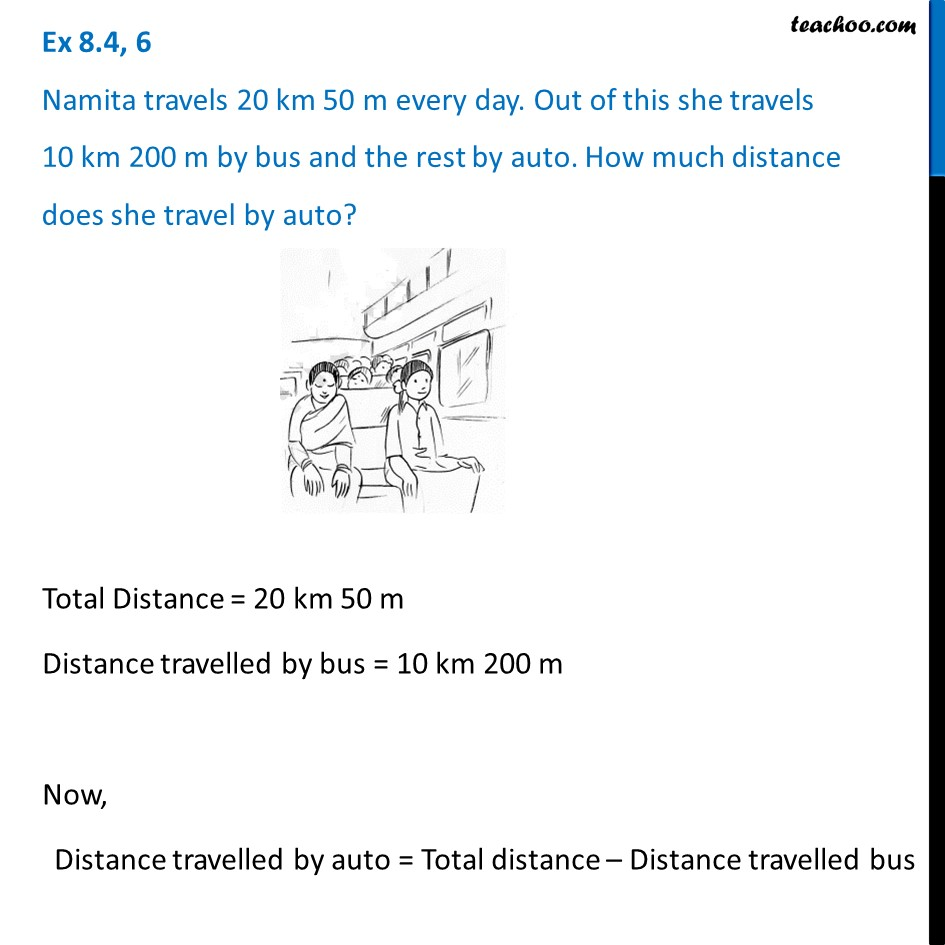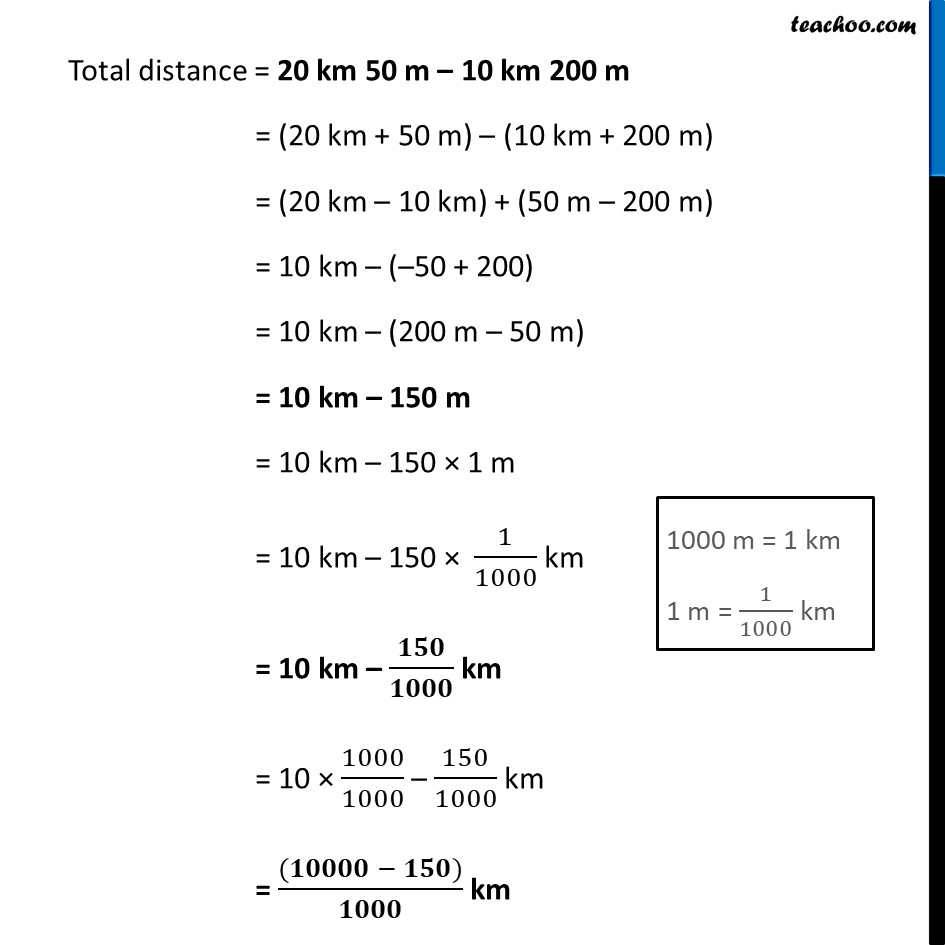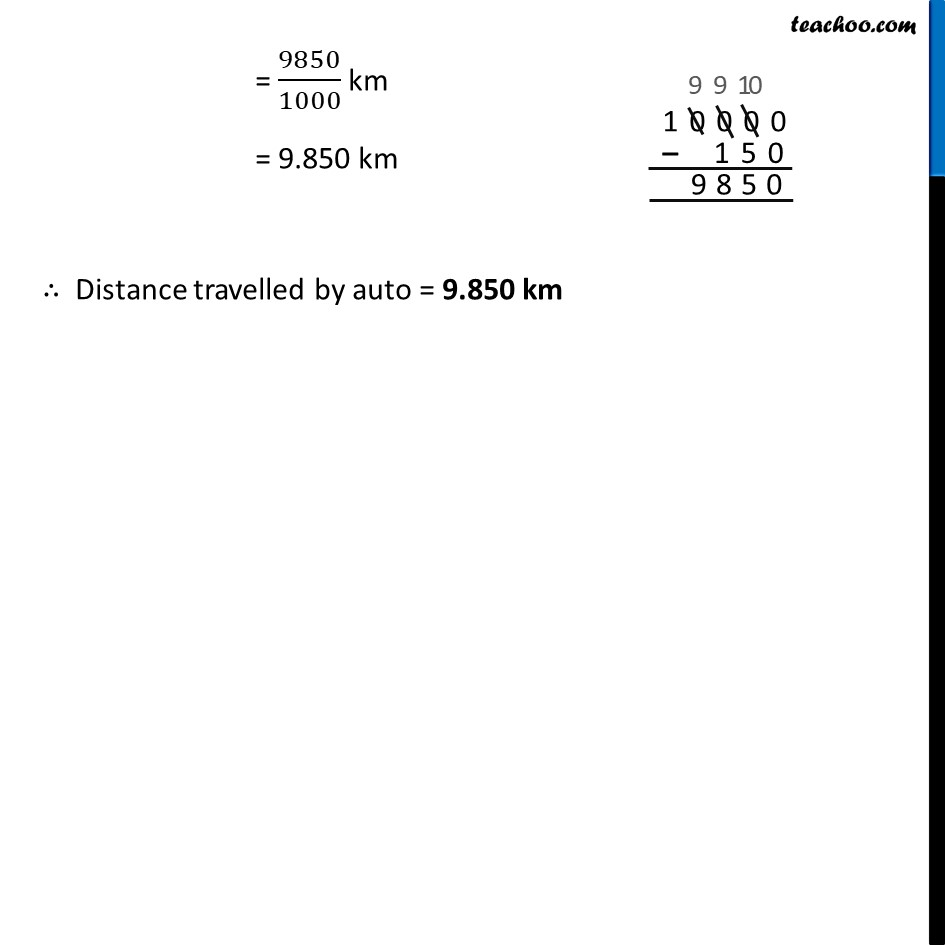Ex 8.4

Chapter 8 Class 6 Decimals
Serial order wiseLearn in your speed, with individual attention - Teachoo Maths 1-on-1 Class

### Transcript

Ex 8.4, 6 Namita travels 20 km 50 m every day. Out of this she travels 10 km 200 m by bus and the rest by auto. How much distance does she travel by auto?Total Distance = 20 km 50 m Distance travelled by bus = 10 km 200 m Now, Distance travelled by auto = Total distance – Distance travelled bus Total distance = 20 km 50 m – 10 km 200 m = (20 km + 50 m) – (10 km + 200 m) = (20 km – 10 km) + (50 m – 200 m) = 10 km – (–50 + 200) = 10 km – (200 m – 50 m) = 10 km – 150 m = 10 km – 150 × 1 m = 10 km – 150 × 1/1000 km = 10 km – 𝟏𝟓𝟎/𝟏𝟎𝟎𝟎 km = 10 × 1000/1000 – 150/1000 km = ((𝟏𝟎𝟎𝟎𝟎 − 𝟏𝟓𝟎))/𝟏𝟎𝟎𝟎 km = 9850/1000 km = 9.850 km ∴ Distance travelled by auto = 9.850 km